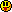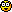# ForumCS2DScriptsDefine the back or the front of player

#Define the back or the front of player

10 replies
Goto Page1KenVo
User
OfflineLet's say if enemies run into the "front"(red area) of the player, then they will get killed. Otherwise nothing happens.
MAX-russia
User
OfflineWhat do you want to say this way ? You need help or what ?
3RROR
User
OfflineDefine what? Sorry I didn't get it.
dENd
User
OfflineLua request?
You want to kill all players in your view line?
© Unreal Software, 2003-2018 | This website is using cookies because they are delicious | Disclaimer | Site Notice
EngiN33R
Moderator
OfflineCode:
1
2
3
4
5
6
7
8
9
10
11
12
13
14
15
16
17
18
19
20
21
22
23
24
25
26
27
28
29
30
31
function findPlyAngle(ply1,ply2)
local x1,y1=player(ply1,"x"),player(ply1,"y")
local x2,y2=player(ply2,"x"),player(ply2,"y")
local ang=-math.deg(math.atan2(x1-x2,y1-y2))
return ang
end

function isInFront(ply1,ply2,f) --ply1 is the player in front of ply2
local ang=findPlyAngle(ply1,ply2)
local rot2=player(ply2,"rot")
if rot2>180 then
rot2=player(ply2,"rot")-180
end
if ang>=rot2-f and ang<=rot2+f then
return true
end
return false
end

function checkforinfront()
for _,id in pairs(player(0,"table")) do
for _,iid in pairs(player(0,"table")) do
if id~=iid then
if isInFront(iid,id,45) then
parse("customkill "..id.." Magic "..iid)
end
end
end
end
end

I guess the thing that actually kills people could be done better, but whatever. I think this is what you asked for. Also the function might not work always.
edited 1×, last 07.04.12 12:09:38 pm
I code, therefore I exist. | Visit my blog for Lua tips and other interesting info
Flacko
User
OfflineI'm guessing you want to know if a point is in the player's field of vision.
This code could work
Code:
1
2
3
4
5
6
7
8
9
10
11
12
13
14
15
16
17
18
19
20
21
if r>math.pi then
r = -math.pi+r%math.pi
elseif r<-math.pi then
r = math.pi-(math.pi-r%math.pi)
end
return r
end
end

local fovw = 120
local fovd = 60
fovconst = math.atan2(fovw/2,fovd)
--fovconst is pi/2 (or 90 degrees)
function isinplayerfov(id,x,y)
local a = math.atan2(player(id,"y")-y,player(id,"x")-x)
local rot = torad(player(id,"rot"))
return (a>(rot-fovconst) and a<(rot+fovconst))
end

I haven't tested it too much so I cant really tell.
Btw, the human FOV isn't exactly 90°, it's actually about 95° according to wikipedia.
To set the exact value set fovconst to 95*math.pi/180
Where 95 is any angle you wish.

Edit: Whoops, 5 minutes too lateEngiN33R
Moderator
OfflineNo,Flacko's code is much better - use it instead.
I code, therefore I exist. | Visit my blog for Lua tips and other interesting info
KenVo
User
OfflineLol thank you! However, Engineer's script look somewhat less complicated to me.
@Those who didn't understand what i was asking forPyKemis
User
OfflineHmm well, I see what you did, it's much easier to see how it works from your script.

Since lua is a learning language it's always best to know what it's doing...however if you're just leeching it doesn't matter.
KenVo
User
OfflineWell I actually wanted to know how it works, because I'm not working on LUA scriptingFlacko
User
OfflineWhoops, seems like I made a big mistake, now it's (hopefully) fixed:
Code:
1
2
3
4
5
6
7
8
9
10
11
12
13
14
15
16
17
18
19
20
21
22
if r>math.pi then
r = -math.pi+r%math.pi
elseif r<-math.pi then
r = math.pi-(math.pi-r%math.pi)
end
return r
end
end

local fovw = 120
local fovd = 60
fovconst = math.atan2(fovw/2,fovd)

function isinplayerfov(id,x,y)
local a = math.atan2(player(id,"y")-y,player(id,"x")-x)
local rot = torad(player(id,"rot"))
local dif = normalizerad(rot-a)
return math.abs(dif)<fovconst
end

To understand how it works it's better to use an image:fovconst is half of the FOV angle
in the script:
Code:
1
fovconst = math.atan2(fovw/2,fovd)

a is the angle from the player to the object
in the script:
Code:
1
local a = math.atan2(player(id,"y")-y,player(id,"x")-x)

The player rot is the player's rotation converted to radians (CS2D uses a bizarre angle system)
in the script:
Code:
1
local rot = torad(player(id,"rot"))

To determine if the target is inside the player's FOV, then the difference between rot and a must be smaller than fovconst
in the script:
Code:
1
2
local dif = normalizerad(rot-a)
return math.abs(dif)<fovconst

normalizerad is just a helper function that makes sure that the angle you pass ranges between [-pi;pi] if it doesn't, then it's transformed. Otherwise, radian operations might become buggy.1﻿